# lua

In programming, operators are symbols or characters that perform specific actions on values or variables. They allow you to manipulate and perform calculations on data in your program.

There are 4 types of operators:
(2) Comparison (greater than, less than, etc.)
(3) Logical (manipulate conditions)
(4) Bitwise (manipulate bits in binary data)

There is one extra operator for concatenation, combining a string with other values into a longer string: (`..`)

``````a = "hello"
b = "world"
print( a .. " " .. b ) --hello world``````###### Arithmetic Operators

These are used to manipulate numbers and perform basic mathematical operations.

 + addition - subtraction * multiplication / division % modulo (get remainder of division) ^ exponentiation (to the power of)
``````--addition
3+2 --5

--subtraction
8-2 --6

--multiplication
7*3 --21

--division
40/5 --6

--modulo
10%7 --3

--exponentiation
4^3 --64
``````###### Comparative Operators

These are used to manipulate values (usually numbers) and perform basic comparisons between two values, usually in an `if` statement.

 == equal to ~= not equal to != not equal to (alternative) < less than > greater than <= less than or equal to >= greater than or equal to
``````if a==b then print("equal") end

if a~=b then print("not equal") end

if a!=b then print("not equal") end

if a<b then print("greater than") end

if a<=b then print("less than or equal") end

if a>=b then print("greater than or equal") end``````

The equal and not equal comparison works with numbers, strings, booleans, and functions.###### Logical Operators

These are used to manipulate or compare values (usually booleans). All logical operators consider both false and nil as false and anything else as true.

###### `and`
 and Returns the first false value it encounters or the last true value if all values are true. It can be used to check multiple conditions at once.
``````--booleans
print( true and true )   --true
print( true and false )  --false
print( false and true )  --false
print( false and nil )   --false
--numbers
print( 10 and 3 )        --3
print( nil and 3 )       --nil
--strings
print( "a" and "b" )     --b
print( "a" and nil )     --nil
``````

or

 or Returns the first true value it encounters or the last false value if all values are false. It can be used to choose between multiple options.
``````--booleans
print( false or false ) --false
print( true or false )  --true
print( false or true )  --true
print( false or nil )   --nil
--numbers
print( 10 or 3 )        --10
print( nil or 3 )       --3
--strings
print( "a" or "b" )     --a
print( nil or "b" )     --b
``````

not

 not Reverses the Boolean value of an expression. It returns "true" if the expression is false and "false" if the expression is true.
``````--booleans
print( not true )    --false
print( not false )   --true
--numbers
print( not -3 )      --false
print( not 0 )       --false
--strings
print( not "b" )     --false
--nil
print( not nil )     --true
``````A common way to use these logical operators is to combine multiple condition checks, for example:

``````if a and b then
print("both true")
end

if a or b then
print("one or both true")
end

if not a and b or c then
print("it's complicated")
end
``````& bitwise and | bitwise or ^^ bitwise xor ~ bitwise not << bitwise shift left >> bitwise shift right >>> bitwise logical right shift <<> bitwise rotate left >>< bitwise rotate right

See Bitwise Operations for more.###### Precedence of Operators

Operator precedence determines the order in which operators are evaluated in an expression. It ensures that expressions are evaluated in a consistent and predictable manner. When multiple operators are used in a single expression, operator precedence helps determine which operators are evaluated first.

#### highest precedence

 ( ) ^ not * / % + - .. << >> ~ | < > <= >= ~= == and or

#### lowest precedence556

14 May 2023

Comments are used to add explanations or notes to your code that do not affect the game's functionality. In other words, code comments are used to provide additional information about the code that will not directly affect how the code runs.

In Lua, comments can be added to the code by using two hyphens (`--`). Anything written after the two hyphens on a line will be ignored when running your game. For example:

``````-- This is a comment in Lua
print("Hello, World!") -- This is also a comment

--[[ use this to create a long
multi-line comment when you
want to explain a lot ]]``````

In the above example, the first line is a comment and will be mostly ignored by PICO-8. Comments do take up cart space as characters, but they do not cost any tokens. The second line will be executed and will output "Hello, World!" to the console. You add a comment at the end of a line of code to make a label or note for that line.

Use multi-line comments ( using `--[[` and `]]` ) to give a long and detailed comment, useful when a single line comment trails off the right side of the screen and you want to make it easier to read without scrolling.

###### Alternative

If you are coming from another programming language and are already familiar with using double slashes ( `//` ) for comments, then you can continue to use them in PICO-8. (This is specific to PICO-8 and not Lua).

``// this is also a single line comment``

Note that this does not work for multi-line comments, nor for naming tabs in the code editor (more on this below).###### Usefulness

Comments can also be used to disable code temporarily during testing or debugging. To do this, you can simply add two hyphens at the beginning of the line of code. This is often referred to as "commenting out" a line of code. For example:

``````print("hello world")
--print("This line of code is temporarily disabled")``````

In the above example, it will print "hello world" but the second print will not be executed because it is commented out.

As a programmer, commenting your code well is just as important of a habit to have as formatting and naming your variables and functions accurately and descriptively.###### Naming Tabs

If you are using the PICO-8 Code Editor, you can name the tabs by placing a single line comment at the top of the tab. These names will be displayed under the tabs as you hover over the tab numbers.

This is great for organizing your code and labeling for ease of navigation between tabs.###### Labeling Carts

To properly label your game cartridge, create 2 single line comments at the very top of your code (in tab #0). These will be displayed below the label section of a .png game cart image. This is usually used to first name the cart, and second name the developer.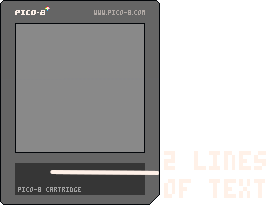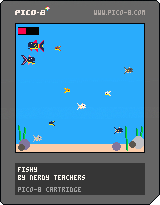354

8 May 2023

The word "condition" means a mode or state of being.
a light can be on or off; you can be happy or sad or mad

The word "conditional" means depending on a mode or state of being.
if the light is off then turn it on; if you are happy then I am happy

In programming, conditionals are exactly like this, but there are only two options: true or false. Writing conditionals is like playing the game 21 Questions with your computer. Just like that game, you can only ask Yes or No questions. However, instead of yes and no, the computer will respond with either `True` or `False`.

## If

Conditionals are used to execute different blocks of code depending on whether a condition is true or false. In other words, conditionals allow you to ask a true or false question, then run certain code only if that is true. We can draw a conditional like this in a flowchart: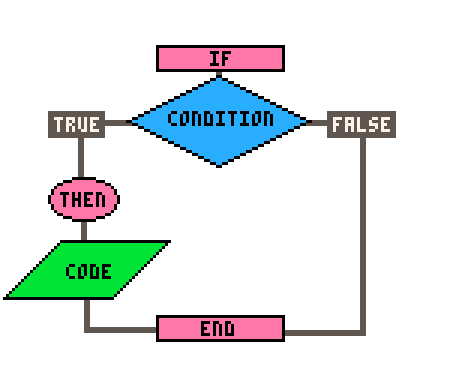In code, this simple conditional is written:

``````if condition then
--code
end
``````

Here's an actual example where we want to know if a variable named `x` is greater than `10`:

``````if x > 10 then
print("x is greater than 10")
end``````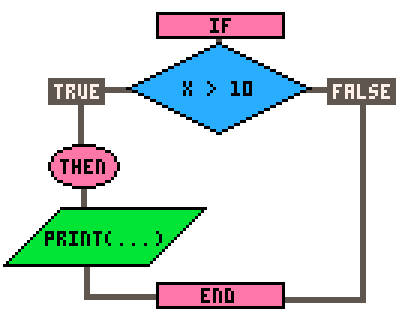In the above example, the "if" statement checks whether the variable "`x`" is greater than 10. If it is, the code inside the block ( in this case, the "`print()`" statement ) will be executed. If the condition is not true, the code inside the block will be skipped and the conditional is closed with `end`.## If Else

You can also use an "`if else`" statement to execute different blocks of code depending on whether a condition is true or false.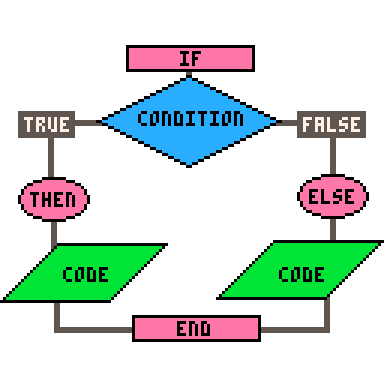Here's an example:

``````if x > 10 then
print("x is greater than 10")
else
print("x is less than or equal to 10")
end``````

In the above example, if "`x`" is greater than 10, the code inside the first block will be executed. If "`x`" is less than or equal to 10, the code inside the second block will be executed.## If Elseif Else

Finally, you can use an "`if elseif else`" statement to test multiple conditions.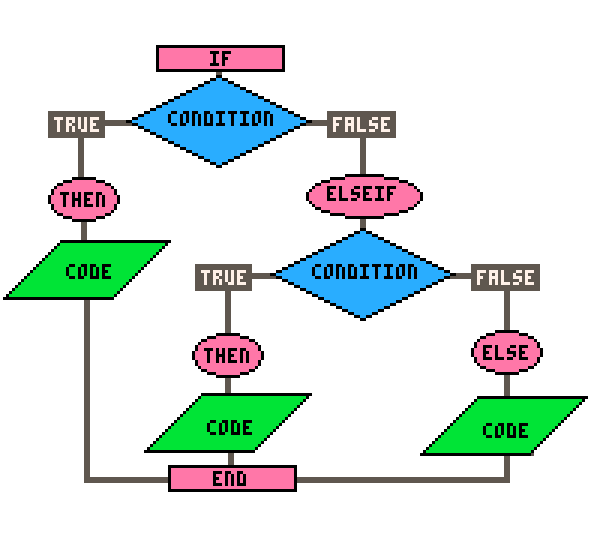Here's an example:

``````if x > 10 then
print("x is greater than 10")
elseif x == 10 then
print("x is equal to 10")
else
print("x is less than 10")
end``````

In the above example, if "`x`" is greater than 10, the code inside the first block will be executed. If "`x`" is equal to 10, the code inside the second block will be executed. If neither condition is true, the code inside the third block will be executed.

You can use as many `elseif` conditionals as you'd like, but keep in mind that long if/elseif statements like that can use a lot of processing power to check every condition before getting to the last one, especially if you run these checks every frame of the game.Here are some tips to keep in mind to make these long conditional statements more readable and efficient:

2. Put the most likely conditions first: This will help evaluate the condition faster and reduce the number of unnecessary condition checks.

3. Limit the number of conditions: If you have too many conditions, consider refactoring your code to use a different approach, such as a loop or a table lookup.

4. Use else to catch all other cases: This will help you avoid unexpected behavior if your conditions do not cover all possible cases.

5. Organize your code into tables or functions: If your if-elseif statements are getting too long, consider breaking up your code into smaller functions. This will help you better organize your code and make it more maintainable.Take note of these things:

`if` is the opening of the statement and requires three things: a condition, followed by a `then`, and closed with an `end`.

- `if` conditions may have parentheses but do not require them, unless using the shorthand form (more below).

- `elseif` is a single word without spaces.

- `elseif` requires a condition followed by a `then` but does not have an `end` for itself.

- `else` does not have a condition, `then`, nor `end` for itself.#### Not for Beginners

Shorthand `if`statement:

``if (condition) code``

Parentheses are required for this, it must be on one line, and must not have `then`, `elseif`, `else`, nor `end`. Here is an example:

``if (x>10) print("x is greater than 10")``

A common usage for this is when you have multiple simple if statements such as checking for button presses. For example:

``````--longform
if btn(0) then x-=1 end
if btn(1) then x+=1 end
if btn(2) then y-=1 end
if btn(3) then y+=1 end

--shorthand
if (btn(0)) x-=1
if (btn(1)) x+=1
if (btn(2)) y-=1
if (btn(3)) y+=1``````

#### (Note: This shorthand does not cost less tokens.)

Shorthand Assignments using `if elseif else` ("ternary operator" in other languages):

``x = condition and a or b``

This will check if the condition is true, then set `X` to `A`, but if the condition is false, then set `X` to `B`.

Warning: This breaks if `A` is `false` or `nil`.

An example of this, while checking for button inputs, could be:

``````--longform
if btn(0) then
player_dx = -1
else
player_dx = 0
end

--shorthand if else
player_dx = btn(0) and -1 or 0
``````

This shorthand form will set a player_dx variable to negaitve one if the player presses button left (#0) or to zero if not pressing button left.

You can even add an elseif secondary check using another pair of `and or` conditionals.

``````--longform
if btn(0) then
player_dx = -1
elseif btn(1) then
player_dx = 1
else
player_dx = 0
end

--shorthand if elseif else
player_dx = btn(0) and -1 or btn(1) and 1 or 0
``````

This shorthand will check if button left (#0) is pressed, then set player_dx to negative one but if button left is false, then it will also check if button right is pressed, then set player_dx to one, but if both are false, then set player_dx to zero.470

8 Sep 2023

In Lua, metatables are tables that can define custom behavior for other tables. Every table in Lua can have a metatable, which is a separate table that is associated with the original table. When an operation is performed on a table and Lua cannot find the operation in the table itself, it will check the metatable to see if it has a function for handling the operation.

For example, let's say you have a table named `tbl`. You can create a metatable, named `mtbl`, for this table like this:

``````tbl = {}
mtbl = {}

setmetatable( tbl, mtbl )``````

Now any operation performed on `tbl` that Lua cannot find will check `mtbl` instead. This allows you to define custom behavior for certain operations.One common use for metatables is to define operators for tables. For example, if you try to add two tables together ( `tbl1+tbl2` ), you will get an error because this is not a default operation, but with metatables you can define what should happen when the `+` operator is used on your table like this:

``````mtbl = {
--__add called when + operator is used
return { x = (a.x+b.x), y = (a.y+b.y) }
end
}

--create tables and set metatables
tbl1 = {x=1, y=2}
tbl2 = {x=4, y=5}
setmetatable(tbl1, mtbl)
setmetatable(tbl2, mtbl)

--add tables uses metatable defined function for +
local tbl3 = tbl1 + tbl2

-- tbl3 is now {x=5, y=7}``````

In this example, we create a metatable `mtbl` that defines the `__add` function, which is called when the + operator is used on tables. The `__add` function returns a new table that is populated with the sum of the `x` and `y` elements of the two tables being added: `a` and `b`.Another use for metatables is to add default values to tables. For example, if your table does not have a key defined, but your code tries to use it, the value of the key will be `nil`, and often error if trying to perform an operation on `nil`. So instead, you can define a metatable that returns a default value of 0 for any key that does not exist in the table:

``````tbl = {}
tbl.foo = 42

print(tbl.foo) -- prints 42
print(tbl.bar) -- prints [nil]
print(tbl.foo+tbl.bar) -- error!

mtbl = {
__index = function(table, key)
return 0
end
}
setmetatable(tbl, mtbl)

print(tbl.bar) -- prints 0
print(tbl.foo+tbl.bar) --prints 42, no error``````

In this example, we create a metatable `mtbl`that defines the `__index` function, which is a function called when Lua tries to access a key in `tbl` that does not exist. The `__index` function simply returns 0 in this case, providing a default value for any non-existent keys.

Overall, metatables are a powerful feature of Lua that allow you to customize the behavior of tables in many ways. However, they can also be a bit tricky to use, so it's important to read the Lua documentation carefully and practice using them to gain a deeper understanding.

Important note from Lua Documentation on Metatables:

"Any table can be the metatable of any other table; a group of related tables may share a common metatable (which describes their common behavior); a table can be its own metatable (so that it describes its own individual behavior). Any configuration is valid."To remove a metatable from a table, set the metatable to nil:

``setmetatable( tbl, nil )``Here are the operations controlled by metatables from the Lua Manual:

 `__add` the addition (+) operation. If any operand for an addition is not a number, Lua will try to call a metamethod. It starts by checking the first operand (even if it is a number); if that operand does not define a metamethod for __add, then Lua will check the second operand. If Lua can find a metamethod, it calls the metamethod with the two operands as arguments, and the result of the call (adjusted to one value) is the result of the operation. Otherwise, if no metamethod is found, Lua raises an error. ` __sub` the subtraction (-) operation. Behavior similar to the addition operation. `__mul` the multiplication (*) operation. Behavior similar to the addition operation. `__div` the division (/) operation. Behavior similar to the addition operation. `__mod` the modulo (%) operation. Behavior similar to the addition operation. `__pow` the exponentiation (^) operation. Behavior similar to the addition operation. `__unm` the negation (unary -) operation. Behavior similar to the addition operation. `__idiv` the floor division (//) operation. Behavior similar to the addition operation. `__band` the bitwise AND (&) operation. Behavior similar to the addition operation, except that Lua will try a metamethod if any operand is neither an integer nor a float coercible to an integer. `__bor` the bitwise OR (|) operation. Behavior similar to the bitwise AND operation. `__bxor` the bitwise exclusive OR (binary ~) operation. Behavior similar to the bitwise AND operation. `__bnot` the bitwise NOT (unary ~) operation. Behavior similar to the bitwise AND operation. `__shl` the bitwise left shift (<<) operation. Behavior similar to the bitwise AND operation. `__shr` the bitwise right shift (>>) operation. Behavior similar to the bitwise AND operation. `__concat` the concatenation (..) operation. Behavior similar to the addition operation, except that Lua will try a metamethod if any operand is neither a string nor a number (which is always coercible to a string). `__len` explathe length (#) operation. If the object is not a string, Lua will try its metamethod. If there is a metamethod, Lua calls it with the object as argument, and the result of the call (always adjusted to one value) is the result of the operation. If there is no metamethod but the object is a table, then Lua uses the table length operation (see §3.4.7). Otherwise, Lua raises an error.ined `__eq` the equal (==) operation. Behavior similar to the addition operation, except that Lua will try a metamethod only when the values being compared are either both tables or both full userdata and they are not primitively equal. The result of the call is always converted to a boolean. `__lt` the less than (<) operation. Behavior similar to the addition operation, except that Lua will try a metamethod only when the values being compared are neither both numbers nor both strings. Moreover, the result of the call is always converted to a boolean. `__le` the less equal (<=) operation. Behavior similar to the less than operation. `__index` The indexing access operation table[key]. This event happens when table is not a table or when key is not present in table. The metavalue is looked up in the metatable of table. The metavalue for this event can be either a function, a table, or any value with an __index metavalue. If it is a function, it is called with table and key as arguments, and the result of the call (adjusted to one value) is the result of the operation. Otherwise, the final result is the result of indexing this metavalue with key. This indexing is regular, not raw, and therefore can trigger another __index metavalue. `__newindex` The indexing assignment table[key] = value. Like the index event, this event happens when table is not a table or when key is not present in table. The metavalue is looked up in the metatable of table. Like with indexing, the metavalue for this event can be either a function, a table, or any value with an __newindex metavalue. If it is a function, it is called with table, key, and value as arguments. Otherwise, Lua repeats the indexing assignment over this metavalue with the same key and value. This assignment is regular, not raw, and therefore can trigger another__newindex metavalue. Whenever a __newindex metavalue is invoked, Lua does not perform the primitive assignment. If needed, the metamethod itself can call rawset to do the assignment. `__call` The call operation func(args). This event happens when Lua tries to call a non-function value (that is, func is not a function). The metamethod is looked up in func. If present, the metamethod is called with func as its first argument, followed by the arguments of the original call (args). All results of the call are the results of the operation. This is the only metamethod that allows multiple results.472

3 Nov 2023

Coroutines are a way to pause and resume the execution of code in Lua and PICO-8.

A function is a reusable piece of code that performs a specific task, but if you exit a function, and want to continue it, then the entire function must run again. Coroutines are similar to functions, but they can be paused and resumed at any point in their execution. This means that you can write code that yields (pauses) and resumes (unpauses) at specific points, allowing you to control the flow of execution in a more fine-grained way.

To create a coroutine, use `cocreate(function)`:

``c = cocreate(f)``

The function passed into `cocreate()` can then call `yield()` to pause running the function and return control to the main program. Usually, you'll see `yield()` in a loop.

To pause a coroutine, use `yield()`, and to resume it from that yielded point, use `coresume()`:

``````function dialog()
print("hello")
yield()               --pause
print("world")
yield()               --pause
print("how are you?")
end

c = cocreate(dialog)

function _update()
if btnp(4) then
coresume(co)
end
end``````

Having functions that can pause and resume where they left off can be incredibly useful in game development, especially for creating game logic that involves complex or time-consuming tasks. Here are a few examples of how coroutines can be used in game development:

1. Animations:
To create complex animations that involve multiple steps or actions. By breaking the animation down into smaller steps and using coroutines to sequence them, developers can create animations that are more realistic and visually impressive.

2. AI behavior: To create more advanced AI behavior for non-player characters (NPCs) in games. For example, a coroutine could be used to make an NPC "look around" periodically, or to make them move to a new location over time.

3. Cutscenes: To create more dynamic and interactive cutscenes in games. By using coroutines to control the camera, animations, and other elements of the scene, developers can create cutscenes that feel more like a part of the game world.

4. Tutorials: To create more engaging and interactive tutorials to teach the rules of your game. Coroutines can automate actions in your game, then pause to explain what is happening, and resume the gameplay after a player acknowledges by pressing the correct button.

To check the current status of a coroutine, use `costatus(c)` :

``status = costatus(c)``

This function will return 3 possible strings:

`"running"` or `"suspended"` or `"dead"`473

6 Apr 2023

``function name_of_function()``

You may want to create your own functions to help organize your code and make your code more efficient.

Here's an example of a simple custom function that adds two numbers together:

``````function addNumbers(num1, num2)
sum = num1 + num2
return sum
end``````

In this function, we're using the `function` keyword to define a new function called `addNumbers`. The function takes two parameters: `num1` and `num2`, which represent the two numbers we want to add together. Inside the function, we're adding the two numbers together and storing the result in a new variable called sum. Finally, we're using the `return` keyword to send the sum variable back to wherever we asked the function to run.

To run this function, we could write some code like this:

``````result = addNumbers(5, 10)
print(result)
--prints 15``````

This code can be written anywhere else inside our game code. We are creating a variable named `result`and at the same time we're calling the `addNumbers` function to run and we pass the numbers `5` and `10` to the function inside of the parentheses. The function then accepts those numbers in the exact order they were given, so that `num1` becomes `5`, and `num2` becomes `10`. This function will then create a variable named `sum`, add `num1` and `num2` together, then return that sum as the result. So it will add `5` with `10`, and save `15` to `sum`. Then it returns `sum` to where we called it.

The returned value from the `addnumbers` function is then saved as `result`. Finally, we're using the print function to display the value of result on the screen.533

4 Nov 2023

Imagine that you're working in a big factory that makes all sorts of things. In this factory, there are lots of different machines that perform specific tasks. For example, there might be a machine that cuts metal, another machine that welds pieces together, and a third machine that paints the finished product.

In the same way, when we're writing code, we often need to perform specific tasks over and over again. Instead of copying and pasting the same code every time we need to do that task, we can create a "machine" called a function that does the job for us. Just like the machines in a factory, a function is designed to do one specific thing really well.

Here's an example. Let's say you're writing a program that needs to measure the area of a piece of wood. Instead of writing out the math formula every time you need to use it, you could create a function that performs the calculation for you. The function might look something like this:

``````function measure_wood(width, length)
area = width * length
return area
end``````

In this function, you're telling the computer to calculate the area of a rectangle using the width and length parameters that are passed in. Once the calculation is done, you're passing the result back to wherever the function was told to run.

Just like a machine in a factory, you can use this function over and over again whenever you need to calculate the area of a rectangle. You don't need to remember the math formula or write it out every time - you can just call the function and let it do the work for you.#### Important Terms Related to Functions

###### API

An API is a collection of built-in functions that are pre-made and ready to use. PICO-8 has many pre-made functions such as `print()`, `map()`, and `music()`. You don't have to build your own function for playing music or drawing your map on the screen.

###### Custom Function

A custom function is any function that you create in your game that is not a part of the PICO-8 API.

See create custom function for how and why you would want to make your own.

###### Parameters

A parameter (often shortened to "param") is any data that your function is expecting to receive. You name the parameters in the parentheses when creating your function.

``function name_of_function(param1, param2)``

(commonly also referred to as "arguments")

###### Arguments

An argument (often shortened to "arg") is any data that you pass to your function. You place arguments inside of the parentheses when you call the function to run.

``name_of_function(arg1, arg2)``

("arguments" and "parameters" are often confused when they are used as synonyms but it is important to understand their difference)

###### Call

When you tell a function to run, this is "calling" the function. You call a function simply by writing the function name and including parentheses. You may need to pass data ("arguments") inside of the parentheses when you call the function. If the function is not expecting any data, then empty parentheses are fine.

``name_of_function()``

###### Return

A function can "return" data back to where it was called. In the same way that you can pass data to a function, the function can then pass data back as well. Use the word `return` then the value or variable you wish to pass back. When a value is returned, you must catch it in a variable at the place where the function was called.

``````--create
function measure_perimeter(width, length)
perimeter = width*2 + length*2
return perimeter
end

--call
my_perimeter = measure_perimeter(3,2)

print(my_perimeter) --prints 10``````

It is also possible to return multiple values. Use commas to separate the values you are returning. Also make sure you use commas to catch those values where you call the function.

``````--create
function measure_both(width, length)
area = width * length
perimeter = width*2 + length*2
return area, perimeter
end

--call
my_area, my_perimeter = measure_both(3,2)

print(my_area) --prints 6
print(my_perimeter) --prints 10``````432

4 Nov 2023

Many programmers use the terms "argument" and "parameter" as synonyms. It is so common that it is generally accepted even though it often leads to confusion or misunderstanding. It is important therefore to know the difference between these two terms and be able to communicate about functions accurately.

###### Parameters

A parameter (often shortened to "param") is any data that your function is expecting to receive. You name the parameters in the parentheses when creating your function. These will then become local variables within the function, holding the values that are passed to the function.

``function f( param1, param2 ) --set function and params``
###### Arguments

An argument (often shortened to "arg") is any data that you pass to your function. You place arguments inside of the parentheses when you call the function to run.

``f( arg1, arg2 )  --call function, pass args``

#### Important to Remember:

- Arguments pass values; Parameters catch and hold them.

- The order of the parameters determines the order of arguments you must follow.

The first function we all learn in any language is how to output text to the screen. In Lua, this is `print()`. And the very first argument we traditionally learn how to pass is the text, "hello world". Like this:

``print("hello world")``

`Print` is the function name.

`( )` parentheses hold the arguments.

`"hello world"` is the first argument, a string.## Passing Multiple Arguments

The print function can take more than one argument, and we can list the arguments inside the parentheses. It is important to know the order that these arguments need to be passed. When you read about how to use pre-built functions ("API") in PICO-8, you'll want to pay attention to the arguments each function needs, found inside the parentheses. For example, here is how we display all of the arguments that the `print` function can take:

``print( string, [x], [y], [color] )``

Notice how some arguments have square brackets "`[]`" around them. This is commonly used in documentation (like this PICO-8 Guide, or the Official Manual) to show optional arguments. So this function will work even if you only pass one argument, the string, which is the only required argument.

Many built-in functions like `print()` have default values to use if you do not pass explicit values in the optional arguments. For example, the `print()` function will use the last cursor position that was set to be the default `x` and `y` parameters, and the last color that was set to be the default `color` parameter. For example:

``````print("h")          --"h" at last cursor and last color
print("e", 5, 3)    --"e" at (5,3) and in last color
print("y", 5, 3, 7) --"y" at (5,3) and in color 7
``````

Sometimes, functions can handle arguments that are given out of order, though this is uncommon. These functions are built specifically to handle the arguments differently based on the number of arguments passed. For example, if `print()` is given only 2 arguments, it will treat the second argument as the color number, instead of as X. For example:

``print("hello", 5) --"hello" at last cursor, in color 5``## Variable Arguments (varargs) ...

Most functions that you will create will have a set number of parameters. But at some point you may want to create a function that is able to recieve a variable amount of arguments. This is called a "variable argument function" or "vararg function". In PICO-8, three dots (`...`) are used to represent a variable number of arguments that can be passed to a function.

From the Lua Manual:

When a Lua function is called, it adjusts its list of arguments to the length of its list of parameters, unless the function is a vararg function, which is indicated by three dots ('...') at the end of its parameter list. A vararg function does not adjust its argument list; instead, it collects all extra arguments and supplies them to the function through a vararg expression, which is also written as three dots. The value of this expression is a list of all actual extra arguments, similar to a function with multiple results.

Varargs are useful when you want to create a function that can accept a varying number of arguments. For example, let’s say you want to create a function that calculates the sum of any number of values. You could use varargs to achieve this:

``````function sum(...)
local args = {...}  --turns varargs list into table
local sum = 0

for i=1, #args do
sum += args[i]
end

return sum
end
``````

Calling this function with varying number of arguments:

``````sum(1,2)        --3
sum(1,2,3)      --6
sum(1,2,3,4)    --10
sum(1,2,3,4,5)  --15
``````

You could pass this function any number of values, and it will add them all together. In the above example, we first turned the list of varargs (`...`) into a table with `local args = {...}`.  You can also handle varargs with the `select()` function. Let's take the exact same function that creates sums above and rewrite it using `select`:

```function sum(...)
local sum = 0

for i=1, select("#",...) do
sum += select(i,...)
end

return sum
end
```

Instead of creating a table, we use `select("#",...)` to get the total number of varargs, then `select(i,...)` to get the value of each argument out of the varargs list.336

14 May 2023

`Font`